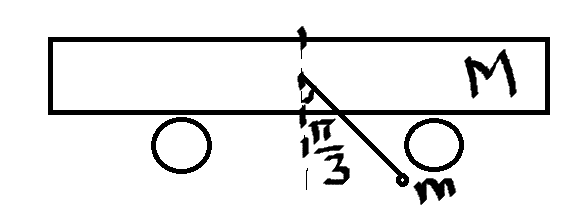# Bob on cart problem

## Homework Statement[Edit: Copy of figure added by moderator]

: http://i.stack.imgur.com/VGhTc.png

(sorry for the shabby figure!)

The problem-a bob of mass ##m## is hanging from a cart of mass ##M##. The system is released from rest from the position shown. Find the maximum speed of the cart relative to ground. String length is ##l##. The answer - ##v= \sqrt{\frac{m^2gl}{M(M+m)}}##

## The Attempt at a Solution

My try- the speed of the cart must be maximum when the bob is at the lowest point relative the the cart. As there is no external force along X-axis, the speed of COM along X axis must be constant. Now, wrt cart, the speed of bob at that point must be ##-\sqrt{gl}## towards left. So, wrt ground, it is ##(v-\sqrt{gl})## towards right, where ##v## is the velocity of cart at that instant relative to ground, which must be the maximum speed, as said earlier. Hence,

##v_{com}=\frac{Mv+m(v-\sqrt{gl})}{M+m}=0##(initially, it was at rest)

Which gives the answer as ##v=\frac{m\sqrt{gl}}{M+m}##.

I don't understand where I have gone wrong. Any help would be appreciated. Thanks in advance!!

ehild
Homework Helper

## Homework Statement

: http://i.stack.imgur.com/VGhTc.png

(sorry for the shabby figure!)

The problem-a bob of mass ##m## is hanging from a cart of mass ##M##. The system is released from rest from the position shown. Find the maximum speed of the cart relative to ground. String length is ##l##. The answer - ##v= \sqrt{\frac{m^2gl}{M(M+m)}}##

## The Attempt at a Solution

My try- the speed of the cart must be maximum when the bob is at the lowest point relative the the cart.
Are you sure? With respect to the ground, the velocities of the car and bob add.
As there is no external force along X-axis, the speed of COM along X axis must be constant. Now, wrt cart, the speed of bob at that point must be ##-\sqrt{gl}## towards left. So, wrt ground, it is ##(v-\sqrt{gl})## towards right, where ##v## is the velocity of cart at that instant relative to ground, which must be the maximum speed, as said earlier. Hence,

##v_{com}=\frac{Mv+m(v-\sqrt{gl})}{M+m}=0##(initially, it was at rest)

Which gives the answer as ##v=\frac{m\sqrt{gl}}{M+m}##.

I don't understand where I have gone wrong. Any help would be appreciated. Thanks in advance!!

@ehild I arrived at that argument considering that when the bob is set into motion, the cart must move in the opposite direction to conserve the momentum. The bob's speed continuously increases till it is at it's lowest point relative to cart, so the cart's speed must also increase in the opposite direction, and hence be maximum when the bob is at the lowest point. Please tell me if I am wrong.

ehild
Homework Helper
I see now, the maximum speed of the cart was the question, and you are right, it is maximum, when the bob is at its lowest position. How did you get the velocity of the bob with respect to the cart there? If you apply conservation of energy you need to take the energy of the cart into account.

I see now, the maximum speed of the cart was the question, and you are right, it is maximum, when the bob is at its lowest position. How did you get the velocity of the bob with respect to the cart there? If you apply conservation of energy you need to take the energy of the cart into account.

I did it this way- If I see the bob wrt the cart, The speed at its bottom most point is ##\sqrt{gl}## by straightforward energy conservation. If I say that the cart itself moves at a speed ##v## at that instant, the absolute velocity of bob at that instant is ##v-\sqrt{gl}## . Am I right?

@ehild Okay I got it...I did it by analysing their rotational motion about their COM....it gives the right answer. Can you point out though the problem with my approach?

Delta2
Homework Helper
Gold Member
Not sure but if you apply conservation of energy and conservation of the x-axis-momentum (take velocities of both wrt the ground), what do you get? Your mistake must be in calculating ##\sqrt{gl}## as the velocity of bob relative to cart, I don't think you apply correctly conservation of energy.

@Delta² I did the exact same thing and got a different answer ( as I mentioned in the question). I believe the relative velocity is right-

## mgl(1-cos\frac{\pi}{3})=1/2mv^2## (relative to cart) which gives ##v=\sqrt{gl}##. So, at that instant, if the cart moves at ##v##, I get bob's absolute speed as ##v-\sqrt{gl}##. I don't see my mistake here. Please point it out; I am clueless.

Delta2
Homework Helper
Gold Member
Well I am not sure at all about this but because the cart is an accelerating frame of reference conservation of energy does not hold.

•cr7einstein
ehild
Homework Helper
I did it this way- If I see the bob wrt the cart, The speed at its bottom most point is ##\sqrt{gl}## by straightforward energy conservation. If I say that the cart itself moves at a speed ##v## at that instant, the absolute velocity of bob at that instant is ##v-\sqrt{gl}## . Am I right?
Conservation of energy is valid for the whole system, the energy of the bob is not conserved. The initial potential energy of the bob transforms into the kinetic energy of the bob and cart. So gm(1-cos(pi/3))l= 1/2 [m vbob2+MVcart2], which does not give vrel = √(gl) for the bob.

•cr7einstein and Delta2
Acknowledged.......Thanks everyone!!